• 用C/C++设计一个算法，把矩阵M化为行最简形梯形矩阵A。矩阵A应该满足以下几个条件： 1)若有零行，则零行应在最下方; 2)非零首元（即非零行的第一个不为零的元素）的列标号随行标号的增加而严格递增; 3)非零首元...
（完整的程序附在文末） 1、问题描述： 用C/C++设计一个算法，把矩阵M化为行最简形梯形矩阵A。矩阵A应该满足以下几个条件： 1)若有零行，则零行应在最下方; 2)非零首元（即非零行的第一个不为零的元素）的列标号随行标号的增加而严格递增; 3)非零首元所在的列除了非零首元外，其余元素全为0; 4)非零首元都为1. 输入是一个文件input.txt 1)第一行会输入样本数k; 2)每个样本的第一行会输入n, m，表示矩阵M是nm维的; 3)接下来的n行里，每一行会输入m个元素，以空格隔开. 输出是一个文件output.txt 2)每个样本的第一行会输出n, m，表示最简形梯形矩阵A是nm维的; 3)接下来的n行里，每一行会输出m个元素，以空格隔开.
2、算法流程： 依据题目描述，我们要写出一个能够依次处理多组数据的、能把任意矩阵化为简化阶梯形形式的程序。考虑到矩阵化为简化阶梯形的过程较为繁琐，所以把化简过程分为将矩阵化为阶梯型、将阶梯型化为简化阶梯型两部分。 1)txt文件的读入和输出的实现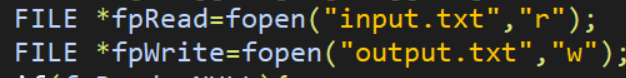2)实现多组数据的处理 为了使程序能处理多组数据，要用到计数循环，即： (1) 输入要处理的数据有多少组，在我的程序中记为k; (2) 定义并赋值k1=1,这里的k1表示正在处理第几组数据; (3) 每处理完一组数据后，使得k1增加1; (4) k1=k时处理完最后一组数据，此时k1再加1，即k1>1，跳出循环.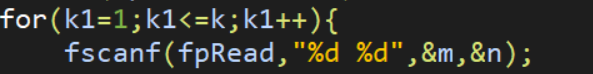3)矩阵的输入 考虑到矩阵包含行和列，是一个二次数列的集合，采用数组记录矩阵会比较方便。这里记为a[m][n]. (1)输入数组的总行数m和总列数n; (2)定义i、j分别表示当前讨论的数是在数组的第i行、第j列(在我的程序中还 定义了i2、j2等参数，即在i、j已经有特定的意义，表示第i行第j列时，进而分析第i2行、第j2列的数。总之，i表示行，j表示列); (3)使i=1，开始一个循环，每次循环结束后i增加1，i>m后跳出循环。在这个循 环中使j=1，开始另一个循环，每次循环结束后j增加1，j>n后跳出循环. (4)在上述循环中，依次从键盘读取a[i][j]. 这样，我们就做到了从上往下、从左往右依次输入数字，使得数字在数组中的位置与在矩阵中的位置完全相同。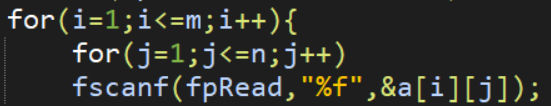以一个3x3，从上往下、从左至右数字依次递增的矩阵为例，我们得到的数组的各个存储格存储的数据如下图：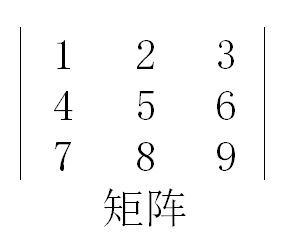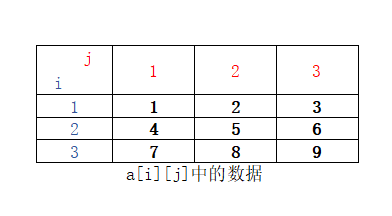4)将一般矩阵化为阶梯型 要想化矩阵为阶梯型，必须从左至右找到每个主元列中的主元，再用主元消去主元正下方的数。首先需要找主元，我采用了类似将矩阵各数字输入数组的类似的方法：用两个循环语句实现：从左至右逐列分析，分析每列时自上而下依次分析每个数字。 (1) 换行 主元位置的数不能为零，所以分析某一列的时候，我们自上而下找第一个不为零的数，让这个数存在的一行与含有该列的主元位置的那一行交换，即换行，这样一来就保证了主元位置不为0。 那么问题来了。若第一列已经处理好，我们要进行第二列的操作时，我们要从第二个数开始自上而下进行判断某个数是否为零。因为若这一列存在主元位置，必然是该列的第二个数。主元位置上方的排序已经完成，不能再动了。那么第三列呢？有两种情况：(a)若第二列找到了主元，第三列有主元位置的话必定是该列第三个;(b)若第二行没有主元，第三列有主元位置的话必定是从上往下数第二个。而我们要从主元位置开始自上而下找非零值，也就是说前面的非主元列会对之后的分析造成影响。经深入思考，我发现当判断到第j列时，用v代表第j列之前有多少非主元列，若第j列有主元，必然是该列第(j-v)个。 但是，我们并不知道某一列是否是主元列，如果不是，这一列不需要进行行交换。如何判断呢？我们自上而下找非零元素，若全部的数均为零，则该列为非主元列，此时v++，并用break跳出循环。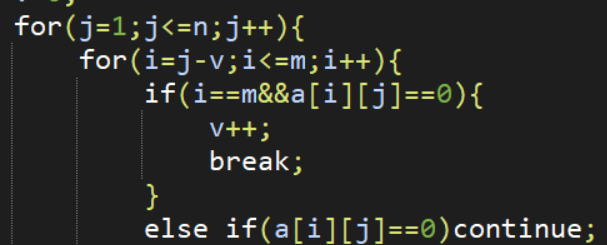换行比较简单，我利用了一个新的数组b来临时存储数据。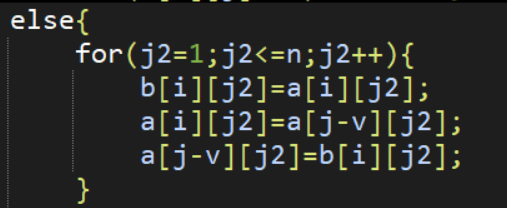(2)利用主元消除该列下方其他行元素 这里我一开始打算将各个主元化为1后再把该列其他数化为0，但是考虑到含有主元的行可能会出现小数，导致之后的处理出现偏差，最终还是决定放大各个数而不是缩小。假设我要用含有某个主元的一行消除第i行，第i行每个数乘以该主元，含有主元的一行乘以主元列中第i行的元素，两行相减赋给第i行。为了避免含主元的一行数据过大，我们在操作结束后除以原来乘的那个数。（因为数据会改变，一开始用一个变量s2来存储这个数。）之后用break跳出循环。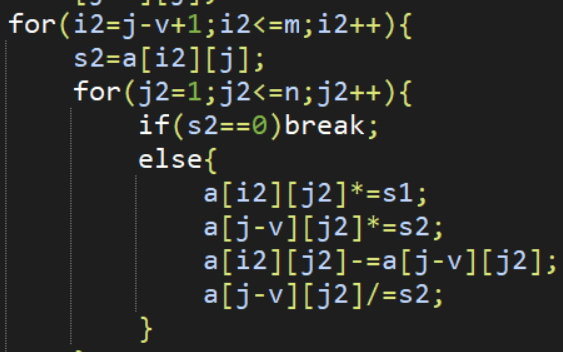至此，我们将矩阵化为了阶梯型。 5)将阶梯型化为简化阶梯型 我们从最后一行开始向上逐行分析，每一行从左至右第一个非零元素必为主元。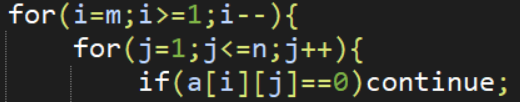将主元化为1，把主元上方的数全部化为0.当然，如果上面的数为零则不处理。 在这里，因为数据处理后会变动，这里利用v记录信息。v等不等于-1是用来记录该数是否为零的一个标准。至此，数据处理全部完成。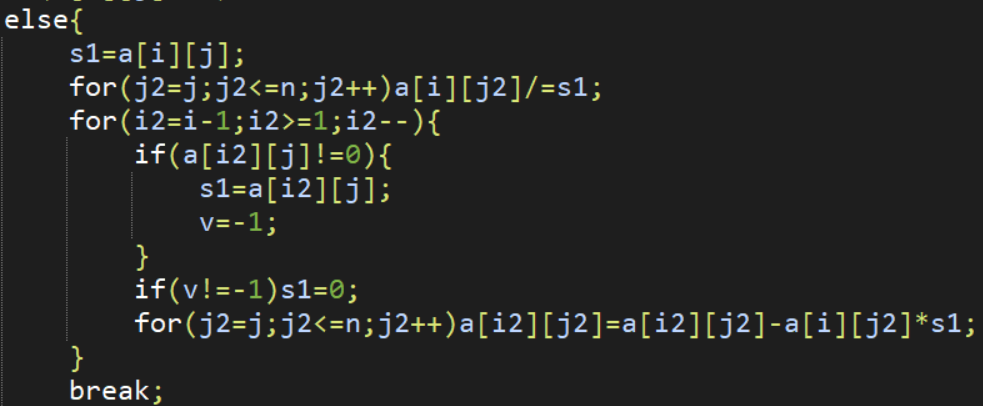6)矩阵的输出。和输入类似，逐行、逐列输出。(保留三位小数)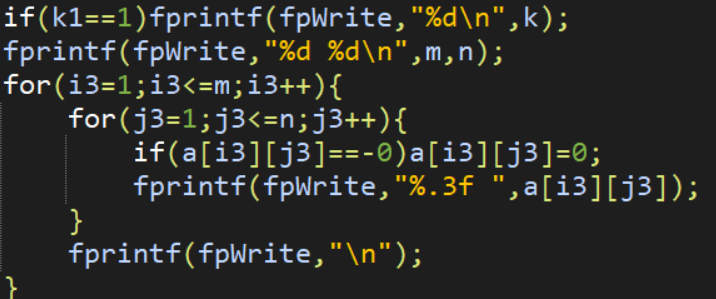3、实验结果 将一个input.txt文件放在与程序相同的文件夹下，运行程序，输出output.txt。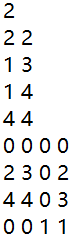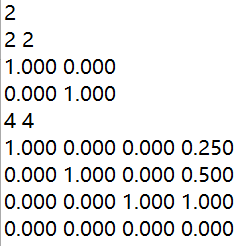在数学里，将一个简单的矩阵化为简化阶梯型很简单，我们的思路也相当明确。但是，当我们要为程序设计一个算法，让程序自己能够用特定的方式解决问题时，却觉得相当的复杂。主元的寻找，一眼就能看出来的，但要让程序做到，不得不绞尽脑汁想出那几行代码。所以，我们要学会探究解决问题的方法的本质。很多显然的事在深究后并不是那么的“显然”，但是一旦我们发现了其中的原理，思想的理解上和之后解决问题的能力上就会有很大的飞越。 完整程序如下：
  #include<stdio.h>
int main(){
int k,k1,m,n,i,j,i2,j2,i3,j3,v,s1,s2;
float a,b;
FILE *fpWrite=fopen("output.txt","w");
return 0;
}
for(k1=1;k1<=k;k1++){
for(i=1;i<=m;i++){
for(j=1;j<=n;j++)
}
v=0;
for(j=1;j<=n;j++){
for(i=j-v;i<=m;i++){
if(i== m&&a[i][j]== 0){
v++;
break;
}
else if(a[i][j]= =0)continue;
else{
for(j2=1;j2<=n;j2++){
b[i][j2]=a[i][j2];
a[i][j2]=a[j-v][j2];
a[j-v][j2]=b[i][j2];
}
s1=a[j-v][j];
for(i2=j-v+1;i2<=m;i2++){
s2=a[i2][j];
for(j2=1;j2<=n;j2++){
if(s2==0)break;
else{
a[i2][j2]*=s1;
a[j-v][j2]*=s2;
a[i2][j2]-=a[j-v][j2];
a[j-v][j2]/=s2;
}
}
}
break;
}
}
}
for(i=m;i>=1;i--){
for(j=1;j<=n;j++){
if(a[i][j]== 0)continue;
else{
s1=a[i][j];
for(j2=j;j2<=n;j2++)a[i][j2]/=s1;
for(i2=i-1;i2>=1;i2--){
if(a[i2][j]!=0){
s1=a[i2][j];
v=-1;
}
if(v!=-1)s1=0;
for(j2=j;j2<=n;j2++)a[i2][j2]=a[i2][j2]-a[i][j2]*s1;
}
break;
}
}
}
if(fpWrite== NULL){
return 0;
}
if(k1== 1)fprintf(fpWrite,"%d\n",k);
fprintf(fpWrite,"%d %d\n",m,n);
for(i3=1;i3<=m;i3++){
for(j3=1;j3<=n;j3++){
if(a[i3][j3]==-0)a[i3][j3]=0;
fprintf(fpWrite,"%.3f ",a[i3][j3]);
}
fprintf(fpWrite,"\n");
}
}
return 0;
}

展开全文c语言
• 利用单纯的矩阵方法得到，两个同型矩阵能够同时化为Hermite标准型的一个充分必要条件是。Rank（A+B）=rankA+rankB。在此基础上，若同型矩阵序列A1，A2…，A1满足，rank（A1+…+A1）=rankA1+…+rankAT，则对任意两个...
• 矩阵标准型进行了，非常详尽的介绍，是自学的理想课件
• 今天我们来聊一聊线性代数中的二次型化为规范形、标准形的内容，这块知识相当重要，我看了看，几乎每一年的考研数学中都会涉及到一道关于这个知识点的题目，这次的整理，不仅帮助大家整理清楚思路，也是为自己整理...

今天我们来聊一聊线性代数中的二次型化为规范形、标准形的内容，这块知识相当重要，我看了看，几乎每一年的考研数学中都会涉及到一道关于这个知识点的题目，这次的整理，不仅帮助大家整理清楚思路，也是为自己整理清楚。
首先，是谈一谈何为二次型
二次型：n个变量的二次多项式称为二次型，就是在一个多项式中，未知数的个数为任意多个，但每一项的次数都为2的多项式。
这个定义给出后，是不是很好理解，未知数的数量随便定，到那时每一项的次数都是2。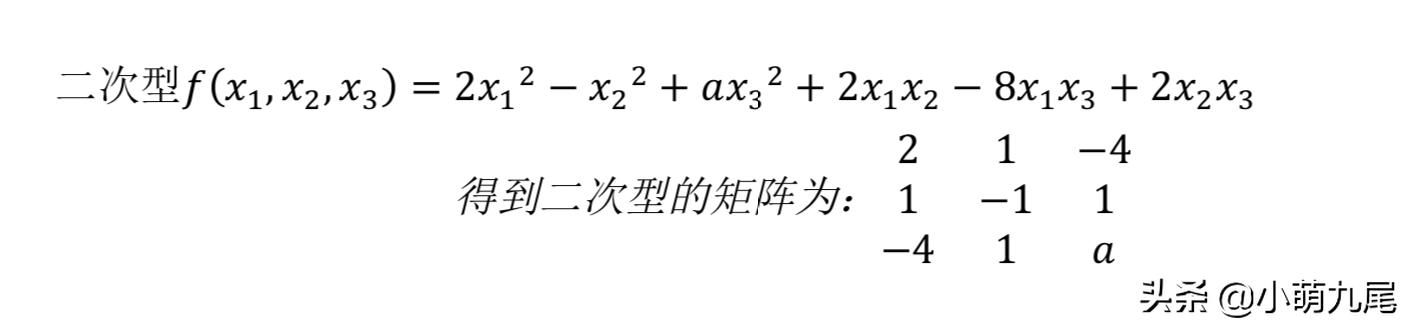如图所示，这就是二次型，可以看到，其中的每一项都是二次，大家有没有注意到我这里写出的二次型矩阵有点奇怪，没错，就是在第一行第二列的值为x1x2的系数一半，这里很重要，当你做题的时候，要用到这个概念之时，便得特别注意写对矩阵，否则若求出特征值和特征向量都会是错误的。
其次，我们来谈一谈标准形和规范形
标准形：如果二次型只有平方项，没有混合项(即混合项的系数全为零)，那么我们就称二次型为标准形，也叫做平方和。
规范形：在二次型的标准形中，如果平方项的系数d只是1,-1,0，就称为是二次型的规范形。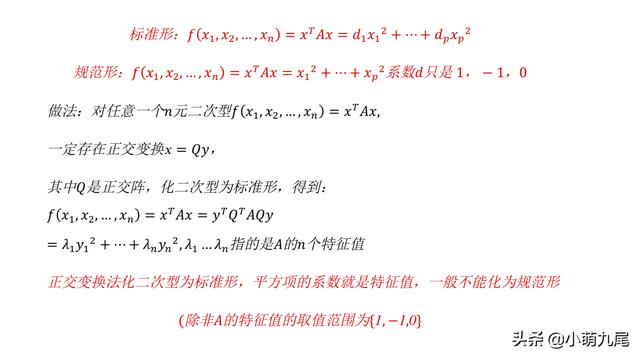给出一道实际例题帮助理解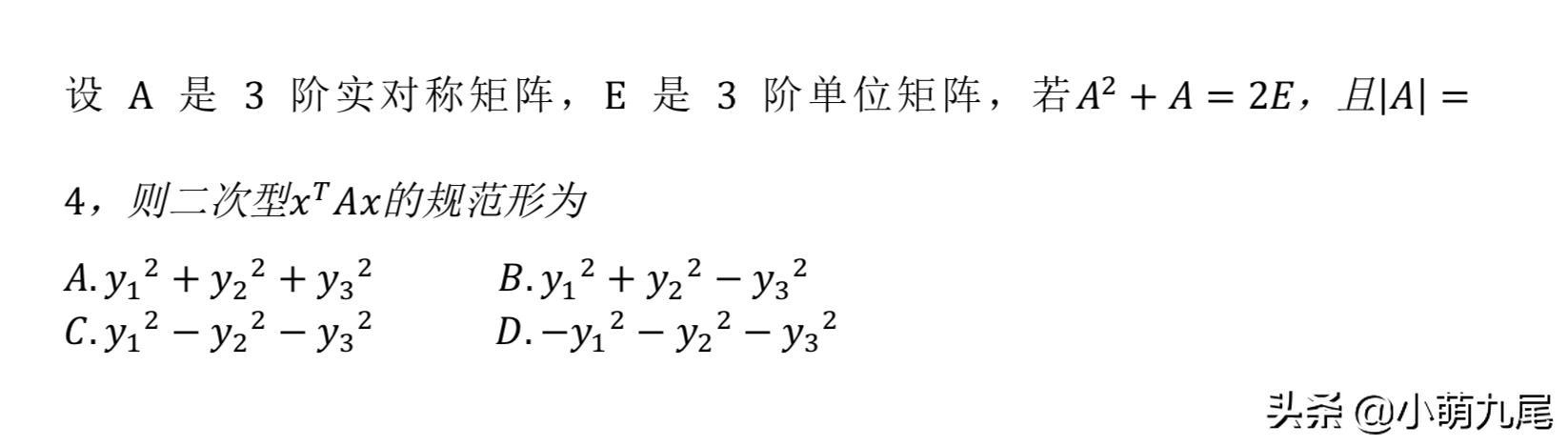我们由规范形的定义可以得知：规范形是由特征值来确定的，因此我们便从特征值入手。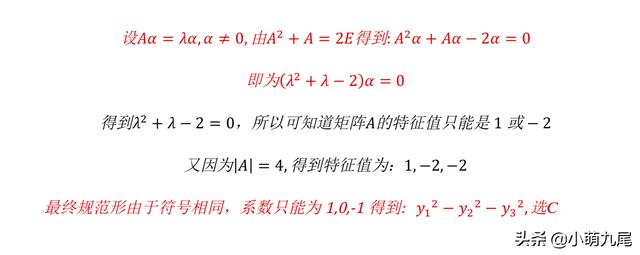正交变换的概念
正交变换：是线性变换的一种，从实内积空间V映射到V自身，且保证变换前后内积不变。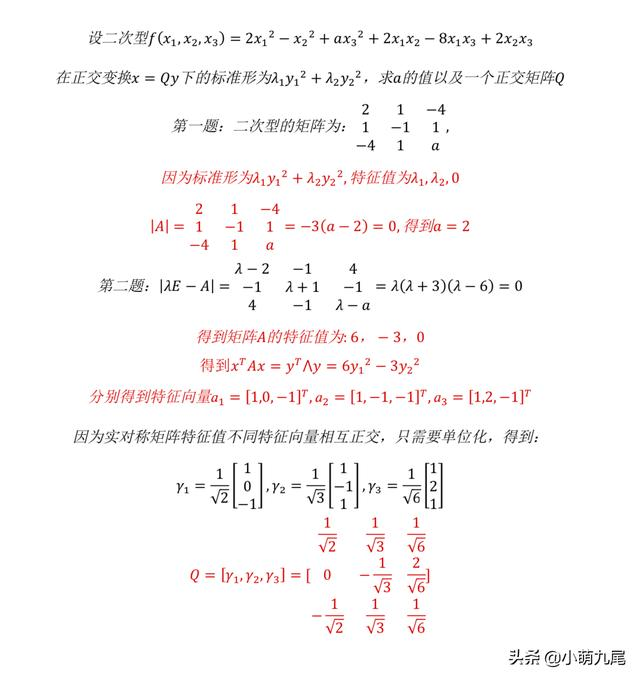总结
总结一下，将二次型化为标准形、规范形，关键在于对基本知识的掌握，难度并不是特别大，要利用好特征值这个概念，对于二次型化为标准形而言，紧紧抓住正交变换不要放，求出特征值，标准形系数便是由特征值构成的。

展开全文• ## 矩阵的 Jordan 标准型

万次阅读 多人点赞 2016-11-24 17:07:27
如果把矩阵化成对角矩阵，关于矩阵的函数计算问题就会大大简化。但一般的矩阵未必与对角矩阵相似。 矩阵标准型有多重，Jordan （约当）标准型是最接近对角矩阵的形式，在控制理论中经常用到。
如果把矩阵化成对角矩阵，关于矩阵的函数计算问题就会大大简化。但一般的矩阵未必与对角矩阵相似。  矩阵的标准型有多重，Jordan （约当）标准型是最接近对角矩阵的形式，在控制理论中经常用到。
存在条件：
设

A∈Cn∗x
$A \in C^{n*x}$, 其特征多项式可以写成如下形式：

φ(λ)=(λ−λ1)m1…(λ−λs)ms

\varphi (\lambda) =  (\lambda - \lambda_1 )^{m_1} \dots  (\lambda - \lambda_s )^{m_s}

其中：

m1+m2+⋯+ms=n
$m_1 + m_2 + \dots + m_s = n$, 那么，矩阵

A
$A$ 可以经过相似变换，化成唯一的 Jordan 标准型 J 。即存在可逆矩阵 P$P$, 满足

P−1AP=J

P^{-1} A P = J

A
$A$ 有Jordan 分解：
A=PJP−1 A = P J P^{-1}

J=diag(J1(λ1),J2(λ2),…,Js(λs))

J = diag( J_1 (\lambda _1),J_2(\lambda _2), \dots, J_s(\lambda _s))

Ji(λi),i=1,2,…,s
$J_i(\lambda _i), i=1,2, \dots,s$ 被称为 Jardon 块。
对应的：

P=(P1,P2,…,Ps)

P=(P_1,P_2,\dots,P_s)

Ji(λi)=diag(J1(λi),J2(λi),…,Jki(λi),)

J_i(\lambda _i) = diag( J_1(\lambda_i), J_2(\lambda_i), \dots,J_{k_i}(\lambda_i), )

Jki(λi),i=1,2,…,ki
$J_{k_i}(\lambda _i), i=1,2, \dots,k_i$ 被称为 Jardon 子块。
对应的：

Pi=(Pi(1),Pi(2),…,Pi(ki)

P_i=(P_i(1),P_i(2),\dots,P_i(k_i)

Jki(λi)=⎡⎣⎢⎢⎢⎢⎢⎢λi00…01λi0…001λi…0……………0001λi⎤⎦⎥⎥⎥⎥⎥⎥∈Cki∗ki

J_ {k_i} (\lambda_i) = \begin{bmatrix}   \lambda _i & 1 & 0 & \dots  & 0\\ 0 &  \lambda _i & 1& \dots & 0\\  0&0 &  \lambda _i  &  \dots  & 0\\     \dots &     \dots & \dots & \dots &  1\\     0 & 0 & 0 & \dots     & \lambda _i   \end{bmatrix} \in C^{k_i * k_i}

求解方法：
1、求矩阵的特征值

λi
$\lambda _i$ 及每个特征值的重数

mi
$m_i$。
计算特征值

λi
$\lambda _i$ 的指标

ki
$k_i$, 即

rank(A−λiI)ki=rank(A−λiI)ki+1
$rank(A- \lambda _i I)^{k_i} = rank(A- \lambda _i I)^{k_i+1}$ 成立的最小正整数

ki
$k_i$,也就是

λi
$\lambda _i$对应的约当块的最大阶数。
2、计算特征值

λi
$\lambda _i$对应的Jardon 块的个数及阶数。

rt=rank(A−λiI)t,t=0,1,2,…,ki

r_t = rank(A- \lambda _i I)^t, t=0,1,2,\dots,k_i

δt=rt−1+rt+1−2rt

\delta _t = r_{t-1} +  r_{t+1} - 2 r_{t}

δt
$\delta _t$ 为

λi
$\lambda _i$对应的

t
$t$  阶约当块 的个数Jt(λi）$J_t ( \lambda _i ）$

3、计算

P
$P$ 矩阵。

先求 Pi,i=1,2,…,s$P_i, i=1,2, \dots,s$ 。
先求

Pit,t=1,2,…,ki
$P_i{t} ,t=1,2,\dots,k_i$
对

t
$t$阶约旦子块，求
(A−λiI)tx=0(A- \lambda _i I )^t x=0  的非零解（唯一）

x
$x$,

Pit=(x,(A−λiI)x,…,(A−λiI)t−1x)P_i{t}=(x,(A-  \lambda _i I )x,\dots, (A-  \lambda _i I )^{t-1}x)
进过组合，就可以得到变换矩阵

P
<script type="math/tex" id="MathJax-Element-7880">P</script>
展开全文控制理论
• ## 二次型化为标准型

千次阅读 2020-09-11 16:37:56
二次型化为标准形有利于我们了解二次型的简单形式、二次型的各种参数如正负惯性指数、得到二次型的规范形、对称矩阵合同的简单形等等。另外，化标准形也是解析几何化简二次曲线和二次曲面的需要。 下面，我们以两...
将二次型化为标准形有利于我们了解二次型的简单形式、二次型的各种参数如正负惯性指数、得到二次型的规范形、对称矩阵合同的简单形等等。另外，化标准形也是解析几何化简二次曲线和二次曲面的需要。
下面，我们以两道题目为例说明计算二次型的标准形的2种方法：
配方法正交变换法
配方法其中这里配方法有无数种配方的方法
正交变换法这里提醒一下就是正交变换法的得到的矩阵只有一个就是特征值组成的矩阵
展开全文•初等因子
• 线性代数之矩阵化成标准型的求法 初等变换法 已知矩阵A 求其该矩阵标准型。 总结 Step1：先对矩阵A仅以初等行变换转换为简化阶梯型 Step2：再实施列变换转换为标准型。 ...
• 本文主要就二次型及其标准型中最基础的概念进行总结归类，这一点是考研中的基础题目，也是一个考研中大家容易忽略的一个板块，有时候容易出现计算错误，大家一定要注意，把这一块练好，希望大家予以重视.定义1.数域K...
• 满意答案zrutj2014.04.11采纳率：59%等级：12已帮助：9169人运用函数eig求出二次型的矩阵A的特征值d和特征向量矩阵v，所求的矩阵d即为系数矩阵A的标准形，矩阵v即为二次型的变换矩阵将下列二次型化为标准型：⑴ ；...
• 矩阵理论研究生学习（一）lambda矩阵lambda矩阵的初等变换行列式因子Smith标准型与不变因子证明题 lambda矩阵 lambda矩阵是含有参数lambda的矩阵，其中有元素是关于lambda的多项式，故又称多项式矩阵。数字矩阵则不...
• 本章包含了：二次型概念 标准型、规范型概念和求解方法二次型转为标准型的三种解法合同变换、合同矩阵、合同关系惯性指数和惯性定理二次型常见的混淆性质本章参考视频系列：厦门大学 高等代数课程 第九章 二次型_...
• 矩阵A、B相似==A、B特征值相同且A、B均可相似标准化(特征对角阵化)——1 矩阵A、B合同==A、B有相同正负惯性指数——2 矩阵A、B均以正交变换进行相似对角化，即A、B均与各自相似标准型合同==A、B与各自对应的二次型，...
• 矩阵的相似标准型之Hamilton-Cayley定理 在该系列第一篇文章中的末尾我们讲到可以利用矩阵的化零多项式来求解矩阵的候选特征解，这一篇文章我们就要讨论矩阵的化零多项式是否一直存在的问题。 一. Hamilton-Cayley...线性代数 化零多项式 特征多项式 数学
• 矩阵的行简化阶梯是一种很有用的与原矩阵等价的矩阵，包括有相同的秩,相同的零空间,以及可以用来求解线性方程组 1 阶梯型矩阵和行简化阶梯型矩阵 下面以上节的方程组开始做初等变换: 由方程组得到增广矩阵 ： ...
• 但是显然 AAA 不会相似于对角矩阵:  \begin{bmatrix} 2 & 0 &0 \\ 0 & 4 & 0\\ 0 & 0 & 2 \end{bmatrix} ⎣⎡​200​040​002​⎦⎤​ 因而只能是相似于若当标准型： J= J = \begin{bmatrix}...特征向量
• 先说一下关于正定矩阵的事情 什么是正定？ 如何判定正定？ 2上标 2下标 但我们还没有讲正定矩阵从何而来 它来自最小二乘法 ATA 假设A是正定的 它的逆是什么？ 它的逆的特征值？ 对称正定矩阵的逆也是对称正定的吗？ ...
•行简化阶梯型 线性代数
• λ1=λ2=1\lambda_1=\lambda_2=1λ1​=λ2​=1求出来的特征矩阵秩为2，有一个特征向量，几何重数是1，小于代数重数2，所以不能相似对角化，只能化成Jordan标准型。 这时候还少一个特征向量，需要找一个特征向量，把...
• ## 用正交变换将二次型化为标准形

万次阅读 多人点赞 2019-04-10 10:47:43
用正交变换二次型化为标准形 用正交变换二次型化为标准形是数学三考研中的重要题型，它综合考察了学生对二次型理论、相似对角化理论、欧式空间理论掌握的熟练程度。解题过程要用到写二次型的矩阵、求矩阵的特征...线性代数 正交变换
•行列式因子 不变因子 初等因子
• ...矩阵在初等行变换下的标准型 ...一、矩阵在初等行变换下的标准型---行标准型 m×n矩阵A= a11 a12 ... a1n a21 a22 ... a2n ... ... ... ... am1 am2 ... amn 的行标准形式是满足一算法 C
• 第十章：矩阵分析10.1. 特征值与特征向量10.1.1. 标准特征值与特征向量问题10.1.2. 广义特征值与特征向量问题10.1.3. 部分特征值问题10.2. 矩阵对角化10.2.1. 预备知识10.2.2. 具体操作10.3. 若尔当(Jordan)标准形...matlab 特征值与特征向量
• Mtrans_uptriangle &... if(is.null(dim(X))|dim(X)==1|dim(X)==1)#不是矩阵时，直接返回本身 { as.vector(X) } else#处理矩阵 { Mrow &lt;- dim(X)#行 Mcol &lt;- dim(X)#列 Mc...
• ## 若尔当标准型求法

万次阅读 多人点赞 2020-11-15 15:36:58
注：定理指出，矩阵的特征矩阵（）一定可以通过初等变换化为上述标准型，称为矩阵标准型。 初等因子：矩阵标准型对角线上次数大于0且首项为1的一次方幂。 本例题中，初等因子为，。 注：虽然上述两个初等因子...线性代数
• %三阶矩阵 A=[0,1,-1;-6,-11,6;-6,-11,5]; B=[0;0;1]; C=[1,0,0]; n=size(A,1); Q1=zeros(n); Q2=zeros(n); J=jordan(A)%约当阵输出 %判断是否能控 for i=0:n-1 Q1(:,i+1)=A^i*B; end n1=rank(Q1); %判断是否...matlab
• ## Jordan 标准型的实例

千次阅读 2017-11-12 14:24:00
将矩阵化为 Jordan 标准型需要三步： 第一步 求出矩阵 $$A \in M_n$$ 全部的特征值 $$\lambda_1,\cdots,\lambda_t$$, 假设有 $$t$$ 个不同的特征值 第二步 Jordan 标准型定理 中的推论告诉我们：\(w_k(A,\lambda)-w...
• 我的微信公众号名称：AI研究...  相似变换是矩阵的一种重要的变换，本章研究矩阵在相似变换下的简化问题，这是矩阵理论的基本问题之一。这种分解简介形式在许多领域中都有重要的作用。   在开始之前说一下矩阵的...
• 化为标准形 f = λ 1 y 1 2 + λ 2 y 2 2 + ⋯ + λ n y n 2 f=\lambda_{1}y_{1}^{2}+\lambda_{2}y_{2}^{2}+\cdots+\lambda_{n}y_{n}^{2} f = λ 1 ​ y 1 2 ​ + λ 2 ​ y 2 2 ​ + ⋯ + λ n ​ y n 2 ​ 其中...线性代数...